SBVS248A November   2015  – November 2015

PRODUCTION DATA.

1. Features
2. Applications
3. Description
4. Revision History
5. Pin Configuration and Functions
6. Specifications
7. Detailed Description
1. 7.1 Overview
2. 7.2 Functional Block Diagram
3. 7.3 Feature Description
4. 7.4 Device Functional Modes
8. Application and Implementation
1. 8.1 Application Information
2. 8.2 Typical Application
3. 8.3 Do's and Don'ts
9. Power Supply Recommendations
10. 10Layout
1. 10.1 Layout Guidelines
2. 10.2 Layout Example
11. 11Device and Documentation Support
1. 11.1 Device Support
1. 11.1.1 Development Support
2. 11.1.2 Device Nomenclature
2. 11.2 Documentation Support
3. 11.3 Community Resources
5. 11.5 Electrostatic Discharge Caution
6. 11.6 Glossary
12. 12Mechanical, Packaging, and Orderable Information

• RTJ|20
• RTJ|20

## 6 Specifications

### 6.1 Absolute Maximum Ratings

over operating junction temperature range and all voltages with respect to GND (unless otherwise noted)(1)
MIN MAX UNIT
Voltage INx, PGx, ENx(3) –0.3 7.0 V
INx, PGx, ENx (5% duty cycle, pulse duration = 200 µs) –0.3 7.5
OUTx –0.3 VINx + 0.3(2)
SS_CTRLx –0.3 VINx + 0.3(2)
NR/SSx, FBx(3) –0.3 3.6
Current OUTx(3) Internally limited A
PGx (sink current into device)(3) 5 mA
Operating junction temperature, TJ –55 150 °C
Storage temperature, Tstg –55 150 °C
(1) Stresses beyond those listed under Absolute Maximum Ratings may cause permanent damage to the device. These are stress ratings only, which do not imply functional operation of the device at these or any other conditions beyond those indicated under Recommended Operating Conditions. Exposure to absolute-maximum-rated conditions for extended periods may affect device reliability.
(2) The absolute maximum rating is VINx + 0.3 V or 7.0 V, whichever is smaller.
(3) Lowercase x indicates that the specification under consideration applies to both channel 1 and channel 2, one channel at a time.

### 6.2 ESD Ratings

VALUE UNIT
V(ESD) Electrostatic discharge Human body model (HBM), per ANSI/ESDA/JEDEC JS-001(1) ±2000 V
Charged device model (CDM), per JEDEC specification JESD22-C101(2) ±500
(1) JEDEC document JEP155 states that 500-V HBM allows safe manufacturing with a standard ESD control process.
(2) JEDEC document JEP157 states that 250-V CDM allows safe manufacturing with a standard ESD control process.

### 6.3 Recommended Operating Conditions

over operating junction temperature range (unless otherwise noted)
MIN MAX UNIT
VINx Input supply voltage range 1.4 6.5 V
VOUTx Output voltage range 0.8 5.0 V
IOUTx Output current 0 1 A
CINx Input capacitor, each input 10 µF
COUTx Output capacitor 10 µF
CNR/SSx Noise-reduction capacitor 1 µF
RPG Power-good pullup resistance 10 100
TJ Junction temperature range –40 125 °C

### 6.4 Thermal Information

THERMAL METRIC(1) TPS7A88 UNIT
RTJ (WQFN)
20 PINS
RθJA Junction-to-ambient thermal resistance 33 °C/W
RθJC(top) Junction-to-case (top) thermal resistance 26.8 °C/W
RθJB Junction-to-board thermal resistance 8.0 °C/W
ψJT Junction-to-top characterization parameter 0.3 °C/W
ψJB Junction-to-board characterization parameter 8.0 °C/W
RθJC(bot) Junction-to-case (bottom) thermal resistance 2.4 °C/W

### 6.5 Electrical Characteristics

over operating temperature range (TJ = –40°C to +125°C), VINx = 1.4 V, VOUTx(TARGET) = 0.8 V, IOUTx = 50 mA, VENx = 1.4 V, COUTx = 10 μF, CNR/SSx = 0 nF, CFFx = 0 nF, SS_CTRLx = GND, PGx pin pulled up to VINx with 100 kΩ, and for each channel (unless otherwise noted); typical values are at TJ = 25°C
PARAMETER TEST CONDITIONS MIN TYP MAX UNIT
VINx(3) Input supply voltage range 1.4 6.5 V
VREF Reference voltage 0.8 V
VUVLO Input supply UVLO VINx rising 1.31 1.39 V
VHYS VUVLO 290 mV
VOUTx Output voltage range 0.8 – 1.0% 5.0 + 1.0% V
Output voltage accuracy(1)(2) 0.8 V ≤ VOUTx ≤ 5 V, 5 mA ≤ IOUTx ≤ 1 A –1.0% 1.0%
ΔVOUTx(ΔVINx) Line regulation IOUTx= 5 mA, 1.4 V ≤ VINx ≤ 6.5 V 0.003 %/V
ΔVOUTx(ΔIOUTx) Load regulation 5 mA ≤ IOUTx ≤ 1 A 0.03 %/A
VDO Dropout voltage VINx ≥ 1.4 V, 0.8 V ≤ VOUTx ≤ 5.0 V,
IOUTx = 1 A, VFBx = 0.8 V – 3%
200 mV
ILIM Output current limit VOUTx forced at 0.9 × VOUTx(TARGET),
VINx = VOUTx(TARGET) + 300 mV
1.5 1.7 1.9 A
IGND GND pin current Both channels enabled, per channel,
VINx = 6.5 V, IOUTx = 5 mA
2.1 3.5 mA
Both channels enabled, per channel,
VINx = 1.4 V, IOUTx = 1 A
4
ISDN Shutdown GND pin current Both channels shutdown, per channel, PGx = (open),
VINx = 6.5 V, VENx = 0.5 V
0.1 15 μA
IENx ENx pin current VINx = 6.5 V, 0 V ≤ VENx ≤ 6.5 V –0.2 0.2 μA
VIL(ENx) ENx pin low-level input voltage (device disabled) 0 0.4 V
VIH(ENx) ENx pin high-level input voltage (device enabled) 1.1 6.5 V
ISS_CTRLx SS_CTRLx pin current VINx = 6.5 V, 0 V ≤ VSS_CTRLx ≤ 6.5 V –0.2 0.2 μA
VIT(PGx) PGx pin threshold For PGx transitioning low with falling VOUTx, expressed as a percentage of VOUTx(TARGET) 82% 88.9% 93%
Vhys(PGx) PGx pin hysteresis For PGx transitioning high with rising VOUTx, expressed as a percentage of VOUTx(TARGET) 1%
VOL(PGx) PGx pin low-level output voltage VOUTx < VIT(PGx), IPGx = –1 mA (current into device) 0.4 V
Ilkg(PGx) PGx pin leakage current VOUTx > VIT(PGx), VPGx = 6.5 V 1 µA
INR/SSx NR/SSx pin charging current VNR/SSx = GND, 1.4 V ≤ VINx ≤ 6.5 V,
VSS_CTRLx = GND
4.0 6.2 9.0 µA
VNR/SSx = GND, 1.4 V ≤ VINx ≤ 6.5 V, VSS_CTRLx = VINx 65 100 150
IFBx FBx pin leakage current VINx = 6.5 V, VFBx = 0.8 V –100 100 nA
PSRR Power-supply ripple rejection f = 500 kHz, VINx = 3.8 V, VOUTx = 3.3 V,
IOUTx = 750 mA, CNR/SSx = 10 nF, CFFx = 10 nF
40 dB
Vn Output noise voltage BW = 10 Hz to 100 kHz, VINx = 1.8 V, VOUTx = 0.8 V,
IOUTx = 1.0 A, CNR/SSx = 1 µF, CFFx = 100 nF
3.8 μVRMS
Noise spectral density f = 10 kHz, VINx = 1.8 V, VOUTx = 0.8 V,
IOUTx = 1.0 A, CNR/SSx = 10 nF, CFFx = 10 nF
11 nV/√Hz
Rdiss Output active discharge resistance VENx = GND 250 Ω
Tsd Thermal shutdown temperature Shutdown, temperature increasing 160 °C
Reset, temperature decreasing 140
(1) When the device is connected to external feedback resistors at the FBx pin, external resistor tolerances are not included.
(2) The device is not tested under conditions where VINx > VOUTx + 2.5 V and IOUTx = 1 A because the power dissipation is higher than the maximum rating of the package. Also, this accuracy specification does not apply on any application condition that exceeds the power dissipation limit of the package under test.
(3) Lowercase x indicates that the specification under consideration applies to both channel 1 and channel 2, one channel at a time.

### 6.6 Typical Characteristics

at TJ = 25°C, 1.4 V ≤ VINx < 6.5 V, VINx ≥ VOUTx(TARGET) + 0.3 V, VOUTx = 0.8 V, SS_CTRLx = GND, IOUTx = 5 mA, VENx = 1.1 V, COUTx = 10 μF, CNR/SSx = 0 nF, CFFx = 0 nF, PGx pin pulled up to VOUTx with 100 kΩ, and SS_CTRLx = GND (unless otherwise noted)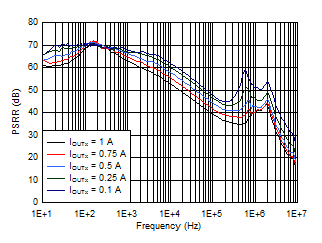VOUTx = 1.2 V, VINx = VENx = 1.7 V, IOUTx = 1 A, COUTx = 10 µF, CNR/SSx = CFFx = 10 nF
Figure 1. Power-Supply Rejection vs Output Current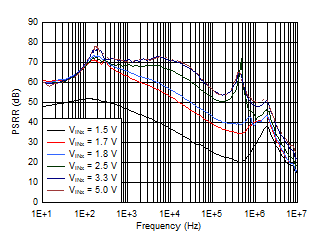VOUTx = 1.2 V, IOUTx = 1.0 A, COUTx = 10 µF, CNR/SSx = CFFx = 10 nF
Figure 3. Power-Supply Rejection vs Input Voltage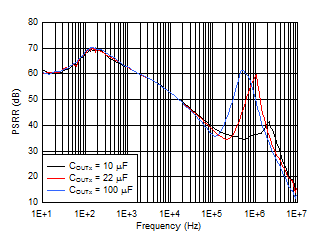VOUTx = 1.2 V, VINx = VENx = 1.7 V, IOUTx = 1.0 A, COUTx = ceramic, CFFx = 10 nF
Figure 5. Power-Supply Rejection vs Output Capacitance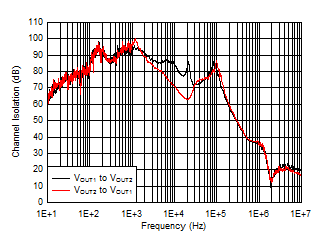VOUTx = 1.8 V, IOUTx = 100 mA, COUTx = 10 µF, CNR/SSx = CFFx = 10 nF
Figure 7. Channel-to-Channel Output Voltage Isolation vs Frequency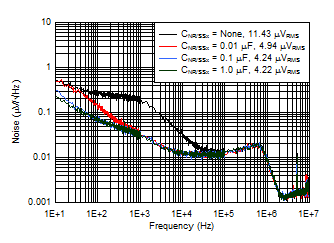VINx = 1.7 V, VOUTx = 1.2 V, IOUTx = 1.0 A, VRMS BW = 10 Hz to 100 kHz, COUTx = 10 µF, CFFx = 10 nF
Figure 9. Spectral Noise Density vs CNR/SSxVOUTx = 1.2 V, IOUTx = 1.0 A, COUTx = 10 µF, CNR/SSx = 10 nF
Figure 11. Spectral Noise Density vs VINx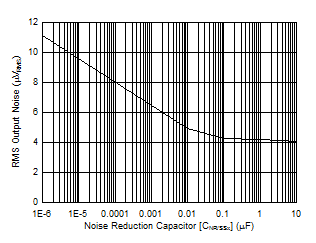VOUTx = 1.8 V, IOUTx = 1.0 A, CFFx = 0.01 µF, BW = 10 Hz to 100 kHz
Figure 13. RMS Output Noise vs CNR/SSx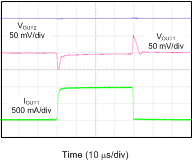VINx = 1.5 V, IOUTx = 100 mA to 1 A to 100 mA at 1 A/µs, COUTx = 10 µF
Figure 15. Load Transient Response (VOUTx = 1.2 V)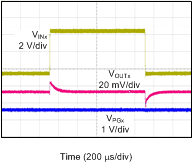VINx = 1.4 V to 6.5 V to 1.4 V at 2 V/µs, VOUTx = 0.8 V, IOUTx = 1 A, CNR/SSx = CFFx = 10 nF
Figure 17. Line Transient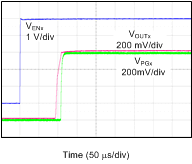VINx = 1.4 V
Figure 19. Start-Up (SS_CTRLx = GND, CNR/SSx = 0 nF)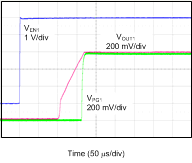VINx = 1.4 V
Figure 21. Start-Up (SS_CTRLx = VINx, CNR/SSx = 10 nF)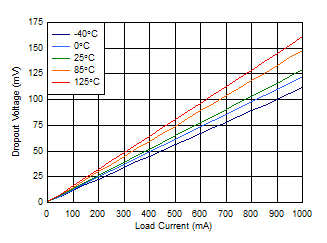VINx = 5.5 V
Figure 23. Dropout Voltage vs Output CurrentVINx = 1.4 V
Figure 25. Load Regulation (VOUTx = 0.8 V)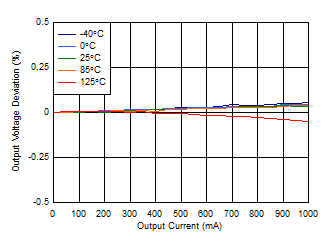VINx = 3.8 V
Figure 27. Load Regulation (VOUTx = 3.3 V)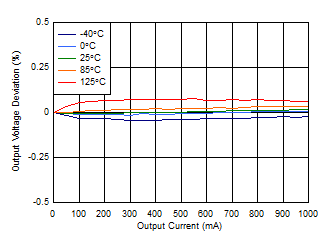VINx = 5.5 V
Figure 29. Load Regulation (VOUTx = 5.0 V)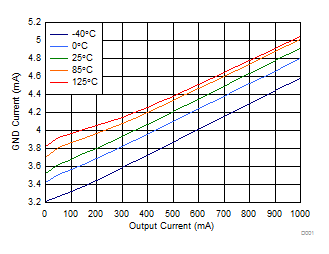VINx = 1.4 V, both channels enabled
Figure 31. Ground Current vs Output Current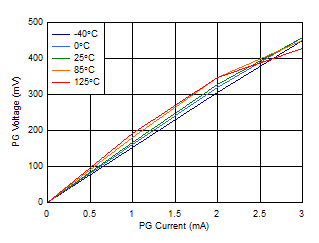Figure 33. PG Low Level vs PG Current (VINx = 1.4 V)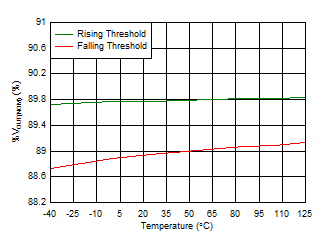VINx = 6.5 V
Figure 35. PG Threshold vs Temperature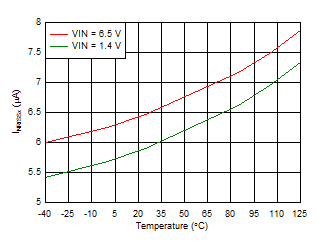Figure 37. Soft-Start Current vs Temperature
(SS_CTRLx = GND)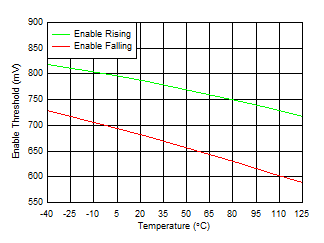Figure 39. Enable Threshold vs Temperature (VINx = 1.4 V)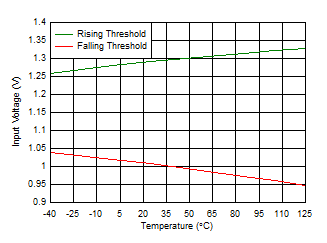Figure 41. Input UVLO Threshold vs Temperature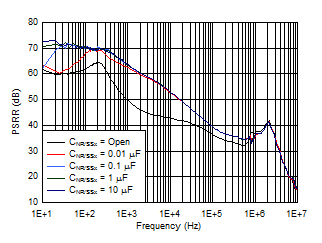VOUTx = 1.2 V, VINx = VENx = 1.7 V, IOUTx = 1.0 A, COUTx = 10 µF, CFFx = 10 nF
Figure 2. Power-Supply Rejection vs CNR/SSx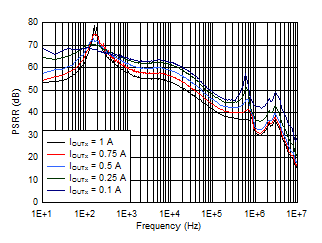VOUTx = 3.3 V, VINx = VENx = 3.8 V, IOUTx = 1 A, COUTx = 10 µF, CNR/SSx = CFFx = 10 nF
Figure 4. Power-Supply Rejection vs Output Current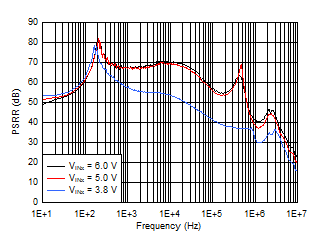VOUTx = 3.3 V, IOUTx = 1.0 A, COUTx = 10 µF, CNR/SSx = CFFx = 10 nF
Figure 6. Power-Supply Rejection vs Input Voltage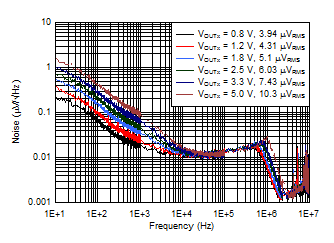VINx = VOUTx + 1.0 V, IOUTx = 1.0 A, VRMS BW = 10 Hz to 100 kHz, COUTx = 10 µF, CNR/SSx = CFFx = 10 nF
Figure 8. Spectral Noise Density vs Output Voltage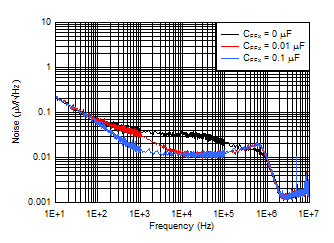VINx = 3.8 V, VOUTx = 3.3 V, IOUTx = 1.0 A, VRMS BW = 10 Hz to 100 kHz, COUTx = 10 µF, CNR/SSx = 10 nF
Figure 10. Spectral Noise Density vs CFFx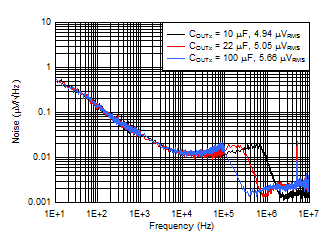VOUTx = 1.8 V, IOUTx = 1.0 A, VRMS BW = 10 Hz to 100 kHz, CFFx = 0.01 µF
Figure 12. Spectral Noise Density vs COUTx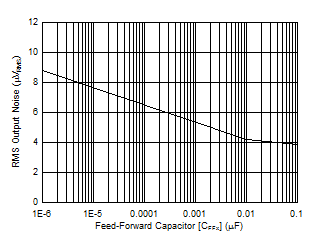VOUTx = 1.8 V, IOUTx = 1.0 A, CNR/SSx = 1 µF, BW = 10 Hz to 100 kHz
Figure 14. RMS Output Noise vs CFFx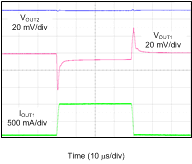VINx = 5.5 V, IOUTx = 100 mA to 1 A to 100 mA at 1 A/µs, COUTx = 10 µF
Figure 16. Load Transient Response (VOUTx = 5.0 V)VINx = 1.8 V, VOUTx = 1.5 V
Figure 18. Current Limit Foldback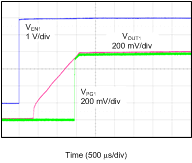VINx = 1.4 V
Figure 20. Start-Up (SS_CTRLx = GND, CNR/SSx = 10 nF)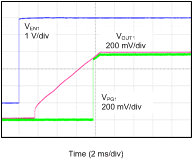VINx = 1.4 V
Figure 22. Start-Up (SS_CTRLx = VINx, CNR/SSx = 1 µF)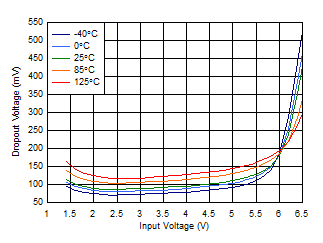IOUTx = 1 A
Figure 24. Dropout Voltage vs Input VoltageIOUTx = 50 mA
Figure 26. Line Regulation (VOUTx = 0.8 V)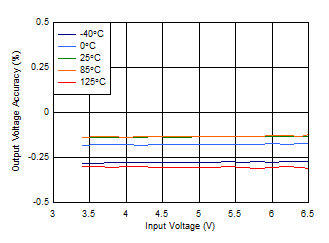IOUTx = 5 mA
Figure 28. Line Regulation (VOUTx = 3.3 V)Both channels
Figure 30. Shutdown Current vs Input Voltage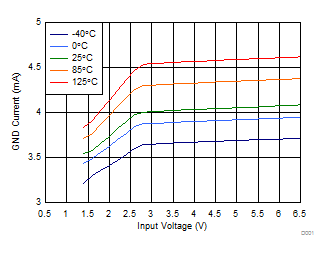Both channels enabled
Figure 32. Ground Current vs Input VoltageFigure 34. PG Low Level vs PG Current (VINx = 6.5 V)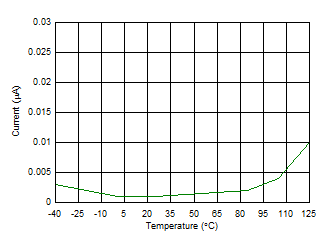VINx = VPGx = 6.5 V
Figure 36. PG Leakage Current vs Temperature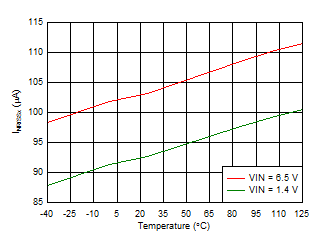Figure 38. Soft-Start Current vs Temperature
(SS_CTRLx = VINx)Figure 40. Enable Threshold vs Temperature (VINx = 6.5 V)